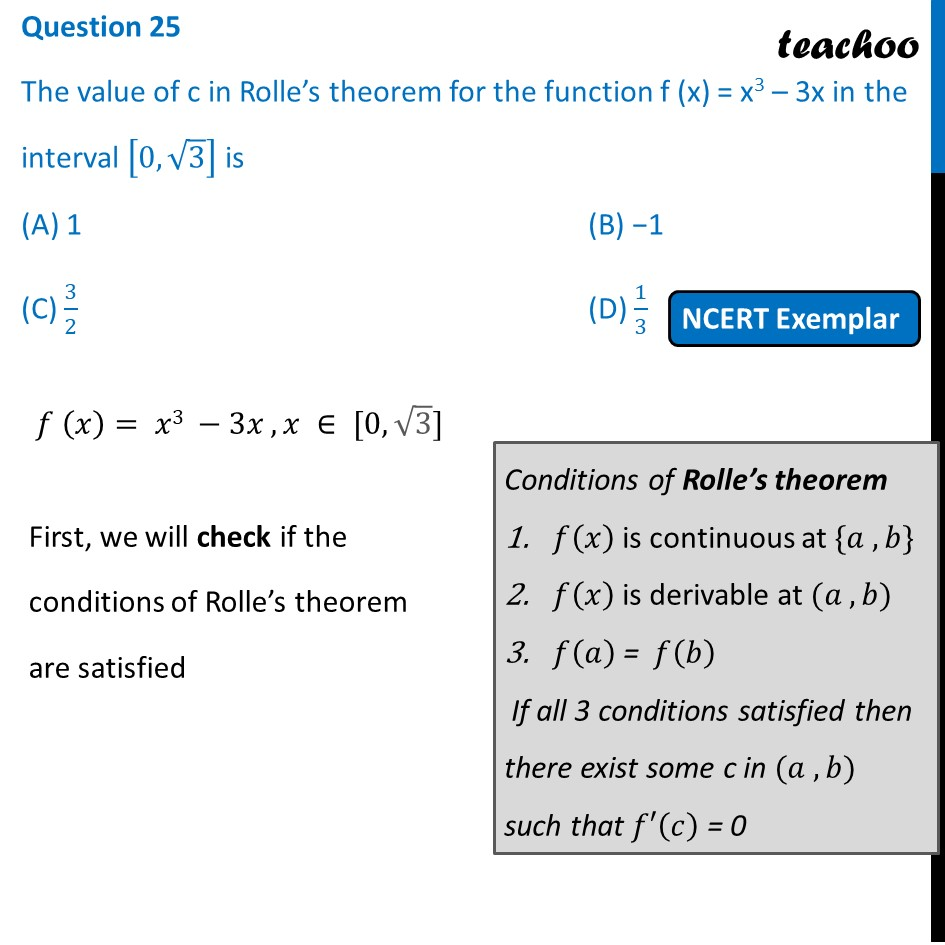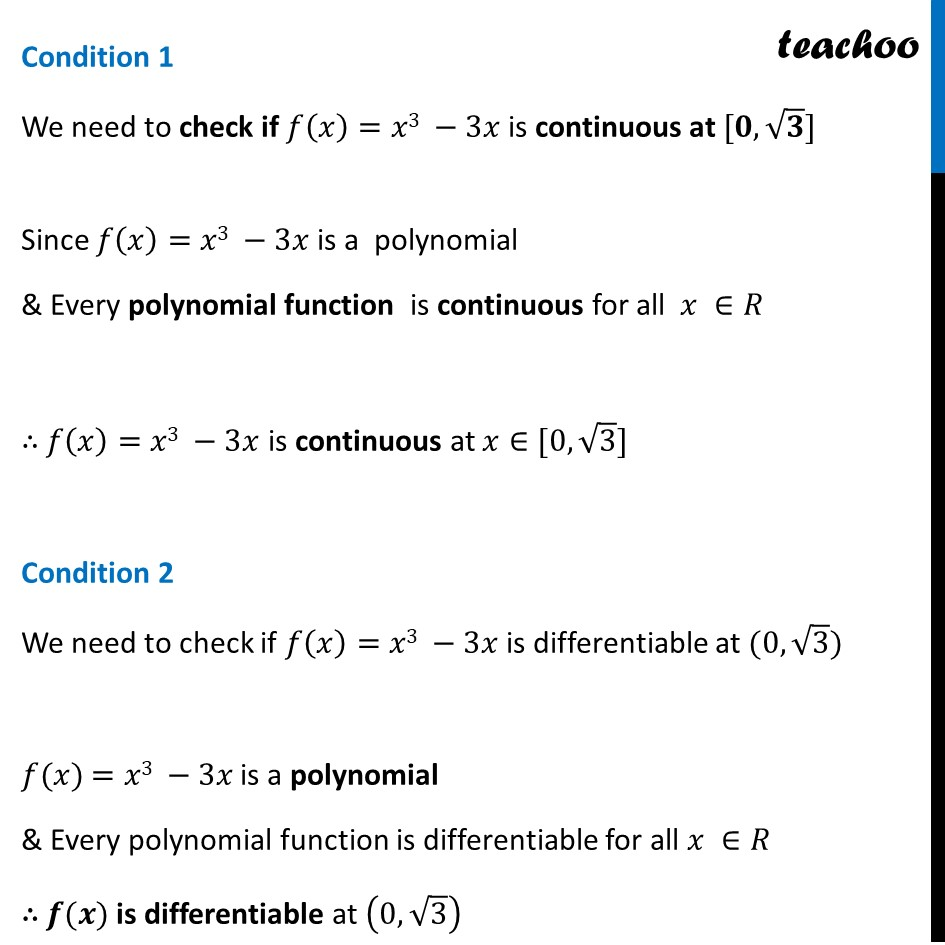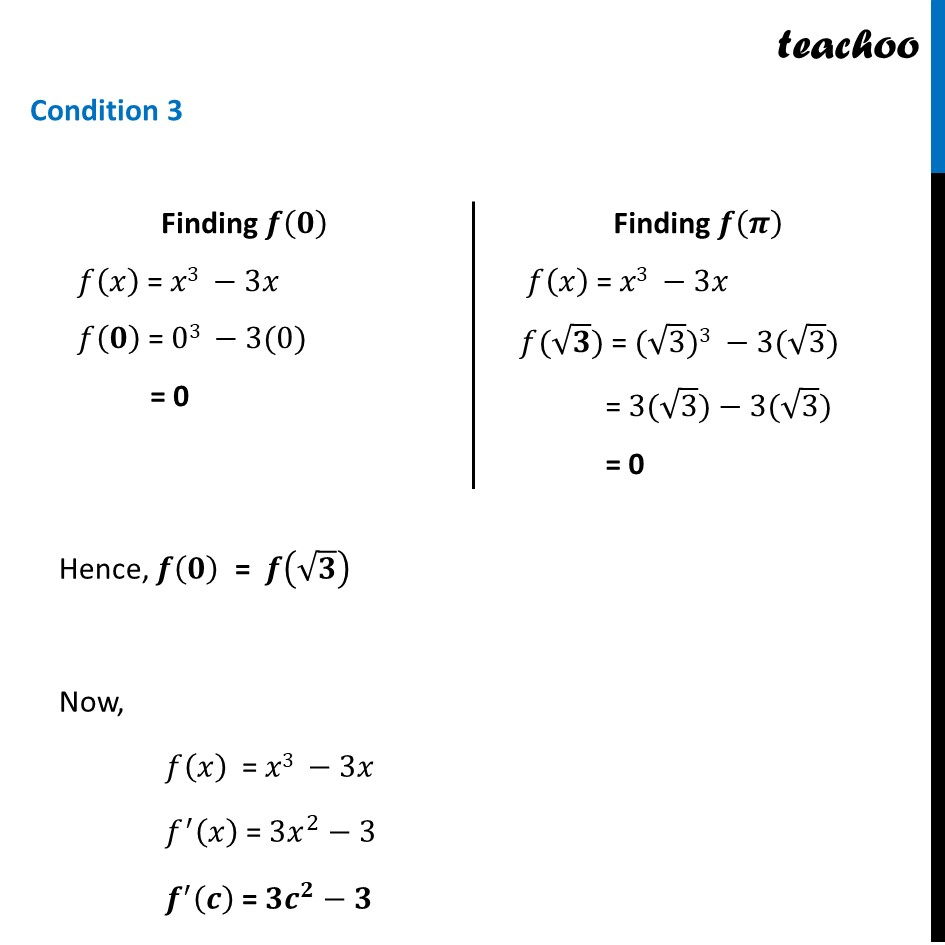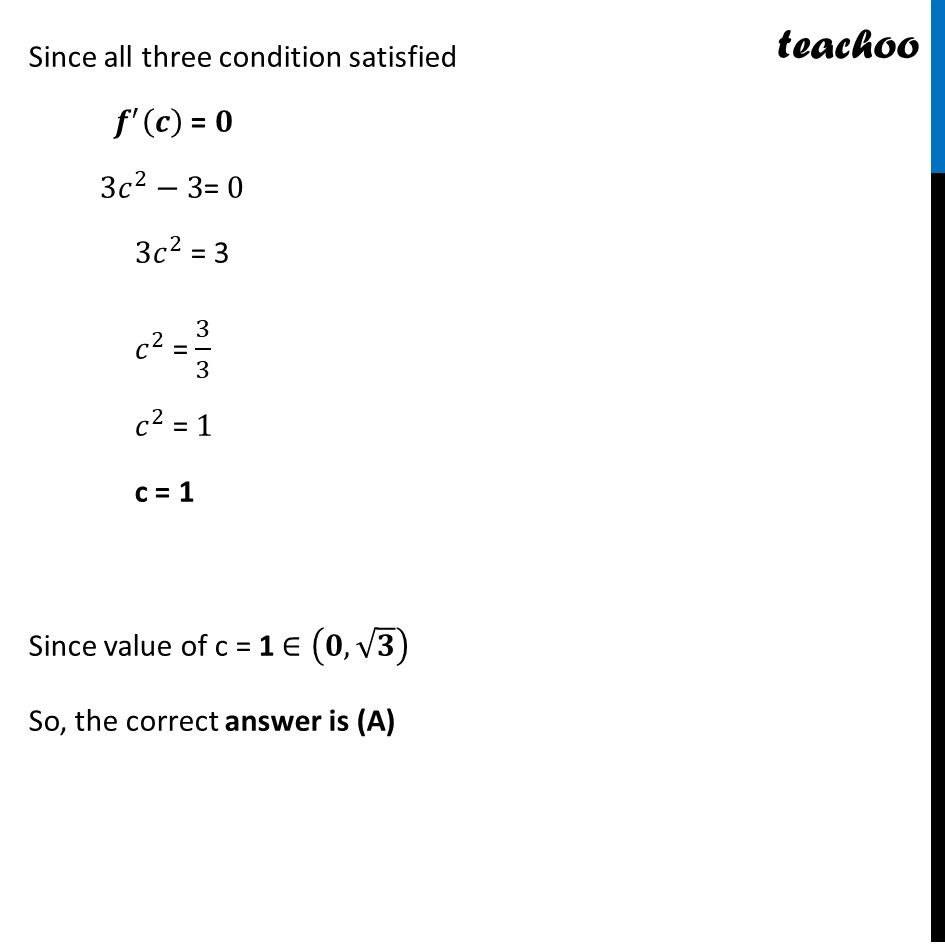NCERT Exemplar - MCQs

Chapter 5 Class 12 Continuity and Differentiability
Serial order wise

## (D) 1/3

This question is similar to Ex 5.8, 1 - Chapter 5 Class 12 - Continuity and DifferentiabilityGet live Maths 1-on-1 Classs - Class 6 to 12

### Transcript

Question 25 The value of c in Rolle’s theorem for the function f (x) = x3 – 3x in the interval [0,√3] is (A) 1 (B) −1 (C) 3/2 (D) 1/3 𝑓 (𝑥)= 𝑥3 −3𝑥 , 𝑥 ∈ [0,√3] First, we will check if the conditions of Rolle’s theorem are satisfied Conditions of Rolle’s theorem 𝑓(𝑥) is continuous at {𝑎 , 𝑏} 𝑓(𝑥) is derivable at (𝑎 , 𝑏) 𝑓(𝑎) = 𝑓(𝑏) If all 3 conditions satisfied then there exist some c in (𝑎 , 𝑏) such that 𝑓′(𝑐) = 0 Condition 1 We need to check if 𝑓(𝑥)=𝑥3 −3𝑥 is continuous at [𝟎,√𝟑] Since 𝑓(𝑥)=𝑥3 −3𝑥 is a polynomial & Every polynomial function is continuous for all 𝑥 ∈𝑅 ∴ 𝑓(𝑥)=𝑥3 −3𝑥 is continuous at 𝑥∈[0,√3] Condition 2 We need to check if 𝑓(𝑥)=𝑥3 −3𝑥 is differentiable at (0,√3) 𝑓(𝑥) =𝑥3 −3𝑥 is a polynomial & Every polynomial function is differentiable for all 𝑥 ∈𝑅 ∴ 𝒇(𝒙) is differentiable at (0,√3) Condition 3 Finding 𝒇(𝟎) 𝑓(𝑥) = 𝑥3 −3𝑥 𝑓(𝟎) = 03 −3(0) = 0 Finding 𝒇(𝝅) 𝑓(𝑥) = 𝑥3 −3𝑥 𝑓(√𝟑) = (√3)3 −3(√3) = 3(√3)−3(√3) = 0 Hence, 𝒇(𝟎) = 𝒇(√𝟑) Now, 𝑓(𝑥) = 𝑥3 −3𝑥 𝑓^′ (𝑥) = 3𝑥^2−3 𝒇^′ (𝒄) = 𝟑𝒄^𝟐−𝟑 Since all three condition satisfied 𝒇^′ (𝒄) = 𝟎 3𝑐^2−3= 0 3𝑐^2 = 3 𝑐^2 = 3/3 𝑐^2 = 1 c = 1 Since value of c = 1 ∈(𝟎,√𝟑) So, the correct answer is (A)